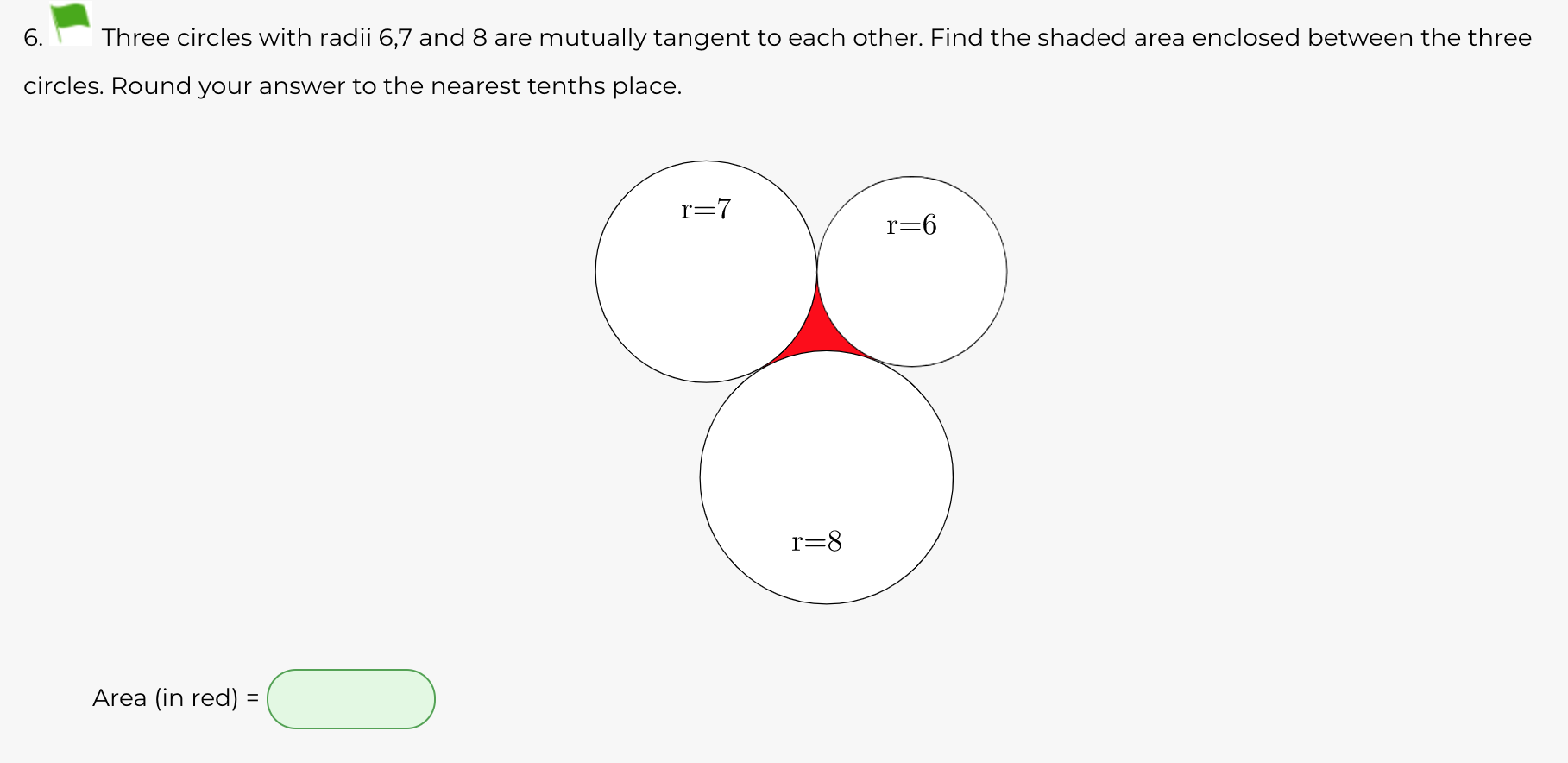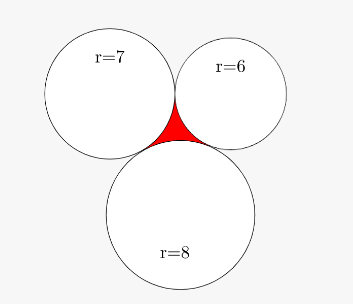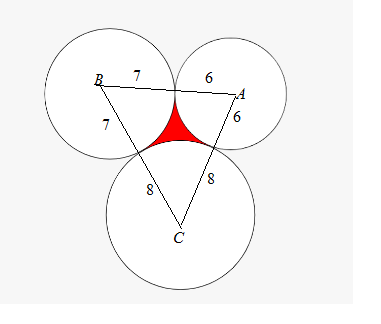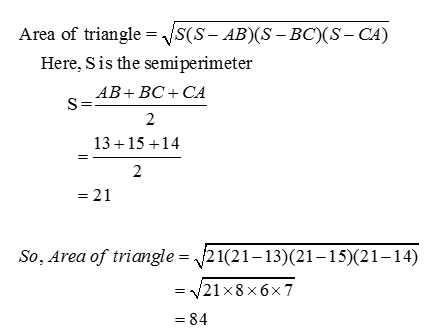# 6.Three circles with radii 6,7 and 8 are mutually tangent to each other. Find the shaded area enclosed between the threecircles. Round your answer to the nearest tenths place.r=7r=6r=8Area (in red) =

Question
27 viewshelp_outlineImage Transcriptionclose6. Three circles with radii 6,7 and 8 are mutually tangent to each other. Find the shaded area enclosed between the three circles. Round your answer to the nearest tenths place. r=7 r=6 r=8 Area (in red) = fullscreen
check_circle

Step 1

Given: Three circle with radii 6,7 and 8 are mutually tangent tot each other.Step 2

Let us make a triangle ABC with vertices at the respective centers of circles.Here, the sides of triangle are AB = 13, BC = 15 and CA = 14.

Step 3...

### Want to see the full answer?

See Solution

#### Want to see this answer and more?

Solutions are written by subject experts who are available 24/7. Questions are typically answered within 1 hour.*

See Solution
*Response times may vary by subject and question.
Tagged in